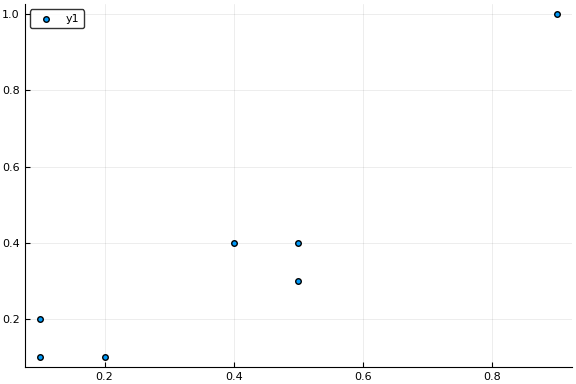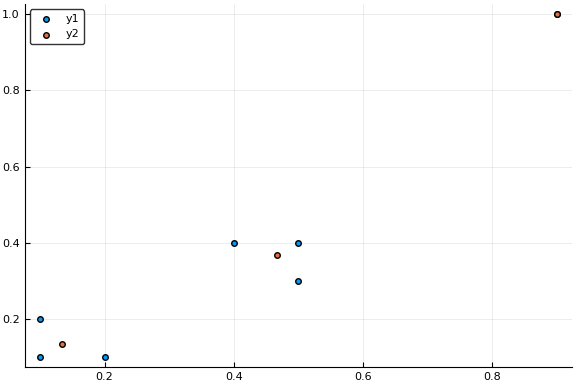### K-means from scratch in Julia

In this post, I’ll attempt to produce a simple implementation of the k-means clustering algorithm in Julia.

The goal of this to gain a better understanding of the underlying process of k-means and provide a minimal, but functioning implementation in Julia.
Please note the code provided here will not be the most efficient, that is not the goal. I’ll try to improve and optimise the code in a later post.
We will assume that we’re given a numerical matrix (A), where each columns of the matrix represent a point in our dataset. We have no assumptions about the number of dimensions of the points inside our dataset, i.e. A can have many rows.
Note: If you’re coming from the word of R or Python, you’re probably used to observations (data-points) being represented as rows. Julia is column-major, meaning it’s more efficient to access points by columns than by rows and this is the reason why we store points as columns. This is common practice in column-major languages such as Julia, Matlab and Fortran.
First, we’ll create some simple test data in just one dimension, so that we can immediately test and debug our code as we go along.
julia> data_simple = [0.1, 0.2, 0.3, 0.7, 0.8, 0.9]'
1×6 RowVector{Float64,Array{Float64,1}}:
0.1  0.2  0.3  0.7  0.8  0.9

I find it easier to define a simple 1D vector and then transpose it with the ' operator to get a 1row x 6 columns matrix. If you eyeball this matrix, you can see 2 clearly visible clusters. The 1st 3 points centred around 0.2 and the last 3 points centred around 0.8. We’ll expect our algorithm to come up with the same answer, when we set k=2.
So let’s revise how k-means works.
1. Initialise the k centroids by picking k points randomly from our dataset.
2. Check each points’ distance from the centroids and assign each point to the closest cluster centroid.
3. Calculate the average of all points per clusters and move the cluster centroids to these points.
4. Go to number 2, iterate until the centroids stop moving.
Sounds simple enough, so let’s start coding.
julia> using StatsBase

julia> function kmeans_simple(X, k, max_iter = 100, threshold = 0.001)

# Let's pick k points from X without replacment
centroids = X[:, sample(1:size(X,2), k, replace = false)]

# create a copy. This is used to check if the centroids are moving or not.
new_centroids = copy(centroids)

# start an empty array for our cluster ids. This will hold the cluster assignment
# for each point in X
cluster_ids = Array{Int32}(size(X,2))

for i in 1:max_iter # I use _ here as we're not using this variable inside the loop
for col_idx in 1:size(X, 2) # iterate over each point

# let's index the ponts one by one
p = X[:, col_idx]

# calculate the distance between the point and each centroid
point_difference = mapslices(x -> x - p, centroids, 1)

# we calculate the squared Euclidian distance
distances = mapslices(sum, point_difference .^ 2, 1)

# now find the index of the closest centroid
cluster_ids[col_idx] = findmin(distances)
# this gives the index of the minimum

# you can uncomment this line to see how the loop progresses
# println("p: $p diff:$point_difference dist: $distances$cluster_ids")
end

# you can uncomment this line to see the internal workings of the funtion
# println("old: $centroids new:$new_centroids")

# Iterate over each centroid
for cluster_id in 1:size(centroids, 2)

# find the mean of the assigned points for that particluar cluster
new_centroids[:, cluster_id] = mapslices(mean, X[:, cluster_id .== cluster_ids], 2)
end

# You can uncomment this line to see how the centers move after each update
# println("old_centroids: $centroids new_centroids:$new_centroids point assignemnts: \$cluster_ids")

# now measure the total distance that the centroids moved
center_change = sum(mapslices(x -> sum(x.^2), new_centroids .- centroids, 2))

centroids = copy(new_centroids)

# if the centroids move negligably, then we're done
if center_change < threshold
# println(i)
break
end
end

# we'll send back both the location of the centroids as well as the cluster ids for each point
return centroids, cluster_ids
end
kmeans_simple (generic function with 3 methods)

Let’s see if this actually works.
julia> kmeans_simple(data_simple, 2)
([0.8 0.2], Int32[2, 2, 2, 1, 1, 1])

So this seems to have done the trick for 1 dimensional data. What about 2D and different k values? It’s always a good idea to try multiple different input values for your functions.
julia> data_complex = [0.1 0.1; 0.1 0.2; 0.2 0.1;  # our first designed cluster
0.4 0.4; 0.5 0.3; 0.5 0.4; # second cluster
0.9 1.0]' # third cluster
2×7 Array{Float64,2}:
0.1  0.1  0.2  0.4  0.5  0.5  0.9
0.1  0.2  0.1  0.4  0.3  0.4  1.0

This looks more complex! Let’s see if our naive little function can handle it.
julia> complex_result = kmeans_simple(data_complex, 3)
([0.9 0.466667 0.133333; 1.0 0.366667 0.133333], Int32[3, 3, 3, 2, 2, 2, 1])

julia> # let's visualise the points
using Plots

julia> scatter(data_complex[1, :], data_complex[2, :])julia> # and add the cluster centroids - red points
scatter!(complex_result[1, :], complex_result[2, :])Success! Now we know more about k-means.
Let’s discuss some of the more obscure functions used in our solution.
point_difference = mapslices(x -> x - p, centroids, 1)
Here we’re using mapslices to apply the anonymous function x-> x-p to the centroids matrix, column-by-column (our last argument is 1). In practice, what we’re doing here is that we take each column of the centroids matrix, i.e. each centroid and take away the current point p from it. Then all is left is to do is to take the sum of the squares of these differences and we get the square of the Euclidean (L2) distance for our point from each cluster. In theory, we should take the square root of this value to get the Euclidean distance, but since square root is monotonic and we’re lazy, we’re happy with the squared distances.
findmin(distances)
findmin, as the name suggest find the minimum of an array. In this case, we want to know which centroid is closest to our beloved point p. findmin actually returns 2 values, the first is the actual value of the minium element and the second is the index of that element – this is what we care about and this is why we select only the second element findmin(distances).
We use very similar calculations, when checking if the centroids moved. Can you spot the slight difference in implementation and why we can do it that way?
So this sums up our simple k-means implementation in Julia. Hope you enjoyed this post, if so please share it with your fellow data people. Also feel free to ask questions in the comments.